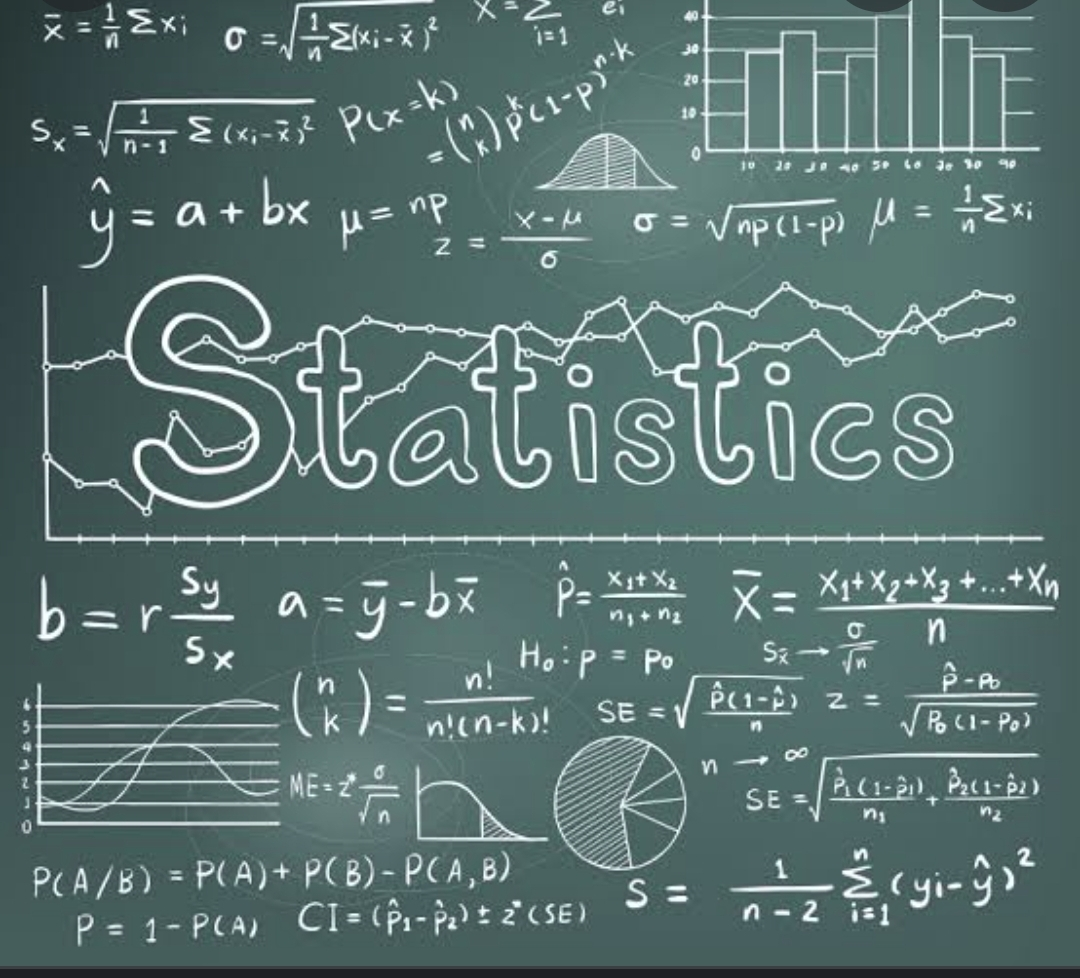Statistics is the practice or science of collecting and analyzing data in large quantities especially for the purpose of inferring proportions in a whole from those in a representative sample.

Statistics is also defined as the branch of mathematics that deals with collecting, organizing, presenting, analyzing and interpreting numerical facts (data) in order to make informed decisions.

There are two branches of statistics namely Inferential and descriptive statistics.
Inferential statistics enables you to draw inference about data. It involves decision making based on data, for example one variable predicts another variable.

Descriptive statistics deals with techniques that are used to organize, summarize and present the data.

Data is defined as raw fact that needs to be processed. Processed data is called information.

Nature of data:

1. Numeric Data: This is also called qualitative data. The data goes beyond appearing as numbers, arithmetic operations can be performed on them. For example, two telephone numbers (09054366743 & 08123466547) cannot be added together. Therefore telephone number does not qualify to be called a numeric data.
Qualitative data can be discrete (whole number) or continuous/ non discrete (decimal).
2. Non numeric data: This is also called quantitative data. Arithmetic operations cannot be carried on non numeric data. It includes policies, categories.

A combination of these two data types is called Mixed data.

Data Timing:

1. Cross sectional data: This is data collected at a particular point in time. For example, February 2022 revenue figures for four companies.
2. Time series or longitudinal data: This is data collected over a period of time. For example, January to March 2022 revenue figures for four companies.
Frequency of data in time series data is how often it is collected.

A combination of cross sectional and time series data is called pool or panel data.

Data is also classified as primary or secondary data based on how it was sourced.

1. Primary data: This data did not exist initially so it is sourced for by the researcher. It is a first hand generated data.
Sources of primary data includes interviews, experiments, focus groups, surveys, observations et.c
2. Secondary data: This is data that already exists. It was generated by a third party such as the government, health facilities, organizations.
Examples of secondary data includes a company’s annual financial report, population census report from the national bureau of statistics (NBS).

The margin of error in a statistical analysis is called level of significance. This is denoted as α.
The confidence level in a statistical analysis is the least percentage of accuracy expected from the experiment. This value varies across different industries. It as denoted as β.
For best practices, a confidence level of 95% is used.

The sum of the confidence level and the level of significance in a statistical analysis is a hundred percent.

An outlier is an observation that lies an abnormal distance from other values in a random sample from a population. The skewness in a normal distribution should be zero.
Outliers can be identified by visual inspection by looking at the minimum and the maximum values of the data set.
A data set that contains an outlier cannot be analyzed using the average value as this will not be reliable, the median value is more accurate in this case.

Mean and standard deviation are used in normal distribution.

Causality in a data set implies that one variable affects another.

Statistics allow you to evaluate claims based on quantitative evidence and help you differentiate between reasonable and dubious conclusions. #MMBA3## Understanding the Balance Sheet and the Income Statement: A Guide for Business Managers #MMBA5

in